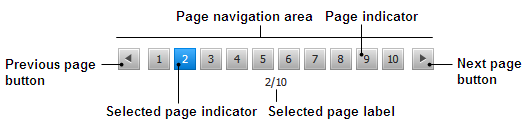# Create a Django Pagination Module

Views: 69
Wrote on April 28, 2020, 8:38 p.m.## Description

Often times, we get involved in building django web apps in which we are required to fetch large sets of data records from the backend, API, or some database sitting somewhere. If you are building a CRM system for example, it could be fetching thousands of customer datasets. If it is a social media app, it could be fetching lots of user comments, profiles, or activities. Whichever it is, there are a couple of methods for handling the data such that it doesn’t become overwhelming to the end-user interacting with the app. At this point, pagination works effectively when you already know the size of the dataset (the total number of records in the dataset) and you only want to load the required chunk of data from the total dataset based on the end-users interaction with the pagination control.

Django has it's own Pagination module, but it requires a little bit of coding on both backend and frontend, especially on the frontend you'll have to add at least 5-10 lines of code to make it customizable for each template you need the pagination to be added..

With this customizable pagination, you only need to setup once by passing three parameters on the backend side and use a shortcut `{{ pagination_html }}` for each of your template.

## Features

• Simply assign the number of shown pagination item by giving a number in setting;
• One shortcut on template, safe marked,, no more line of code.
• Ten Items per page, five shown paging items by default.

## Intruction

1, Save `jw_pagination.py` into your `utils` folder or wherever you want

2,

``````from jw_pagination import JWPagination
``````

3, setup in `views.py`

``````page_linkpath = request.path # required
page_number = request.GET.get('page', 1) # required
total_customer_count = Customer.objects.all().count() # required

search_string = request.GET # this is needed if you have search function
per_page_number = 10 # optional, 10 by default
side_page_number = 2 # optional, 2 by default
``````

4,

``````page_obj = JWPagination(page_number, total_customer_count, page_linkpath, search_string)
pagination_html = page_obj.pagination_html

# Slice operation to get data for each page
customer_objs = Customer.objects.all()[page_obj.data_range_start:page_obj.data_range_end]
``````

## Source code

``````# -*- coding: utf-8 -*-
"""
Created by @JiayuWang on 4/28/20 3:43 PM.
"""
__author__ = '@JiayuWang'

from django.utils.safestring import mark_safe

class JWPagination():

def __init__(self, page_number, total_customer_count, page_linkpath, search_string=None, per_page_number=10, side_page_number=2):
# PARAMS：
# page_number = request.GET.get('page', 1)
# total_customer_count = customer_objs = Customer.objects.all().count()
# search_string = request.GET.copy()
# per_page_number = 10
# side_page_number = 2

try:
page_number = int(page_number)
except Exception:
page_number = 1
if page_number < 1:
page_number = 1

self.total_customer_count = total_customer_count
self.per_page_number = per_page_number
self.search_string = search_string.copy()

_q, _r = divmod(total_customer_count, per_page_number)

if _r:
total_page_count = _q + 1
else:
total_page_count = _q

self.total_page_count = total_page_count

if not total_page_count:
total_page_count = 1

if page_number > total_page_count:
page_number = total_page_count

self.page_number = page_number

start_page_number = page_number - side_page_number
end_page_number = page_number + side_page_number

if start_page_number < 1:
start_page_number = 1
end_page_number = side_page_number * 2 + 1
if end_page_number > total_page_count:

start_page_number = total_page_count - (side_page_number * 2 + 1) + 1
if start_page_number < 1:
start_page_number = 1

end_page_number = total_page_count

self.start_page_number = start_page_number
self.end_page_number = end_page_number
self.total_page_count_range = range(start_page_number, end_page_number + 1)

@property
def data_range_start(self):
return (self.page_number - 1) * self.per_page_number

@property
def data_range_end(self):
return self.page_number * self.per_page_number

@property
def pagination_html(self):

pagination_html = ''

page_pre_html = '<nav aria-label="Page navigation example"><ul class="pagination">'
if self.start_page_number > 1:

self.search_string['page'] = self.page_number-1

pagination_html += page_pre_html

for i in self.total_page_count_range:

self.search_string['page'] = i

if i == self.page_number:
else:

page_next_html = '</ul></nav>'
if self.end_page_number < self.total_page_count:

self.search_string['page'] = self.page_number+1

page_next_html = '<li><a href="{0}?{1}" aria-label="Next"><span aria-hidden="true">&raquo;</span></a></li></ul></nav>'.format(self.page_linkpath, self.search_string.urlencode())

pagination_html += page_next_html

return mark_safe(pagination_html)
``````